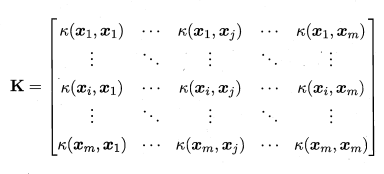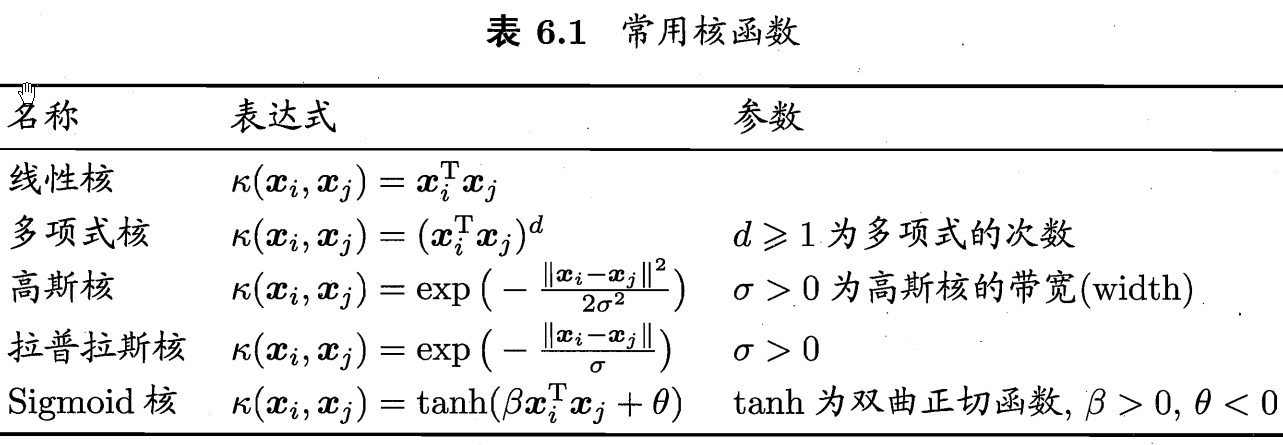# SVM中的核函数

hit2015spring $\frac{hit2015spring}{晨凫追风}$

minw,b12w2s.t.yi(wTϕ(xi)+b)1,i=1,2,3,,m

maxαi=1mαi12i=1mj=1mαiαjyiyjϕ(xi)Tϕ(xj)s.t.i=1mαiyi=0αi0,i=1,2,,m

k(xi,xj)=ϕ(xi),ϕ(xj)=ϕ(xi)Tϕ(xj)

maxαi=1mαi12i=1mj=1mαiαjyiyjk(xi,xj)s.t.i=1mαiyi=0αi0,i=1,2,,m

f(x)=wTϕ(x)+b=i=1mαiyik(xi,x)+b

对称函数## 福利答谢大家！07-12160
06-081万+07-262530
03-288776
04-072万+
04-172万+
04-251万+
04-282万+